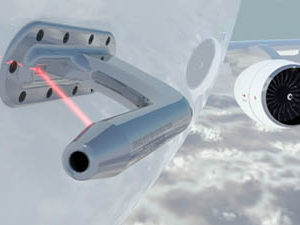# What is a Pitot Tube and How does it Work?Pitot tubes are small, right angle shaped tubes that can measure the velocity of a fluid.  They are an important part of an airplane, where they are used to provide the pilot with in-flight velocity measurements.

When the fluid enters the pitot tube, it is forced to come to a stop.  At some point in the tube, the fluid becomes stationary and all of the kinetic energy possessed by the fluid is transformed into pressure energy.

Bernoulli’s equation can be used to calculate the pressure at the stagnation point, which can then be converted into kinetic energy to find the velocity of the flow.  If you recall, Bernoulli’s principle stated that a finite volume of fluid will have the same amount of energy; It will be neither created nor destroyed.  Thus, a pitot tube is an instrument which conveniently isolates the kinetic energy of a moving fluid.

The kinetic energy of the moving fluid is equal to the pressure energy within the tube.  The pressure within the tube is measured, and the only other variable required is the density of the fluid which is usually known.  If point 1 is outside the tube, and point 2 is somewhere past the stagnation point where the velocity is zero:

Kinetic Energy at Point 1 = Pressure Energy at Point 2$\newline \frac{v_1^{2}}{2} = \frac{p_2}{\rho}\newline v_1 = \sqrt{\frac{2p_2}{\rho}}$

This site uses Akismet to reduce spam. Learn how your comment data is processed.